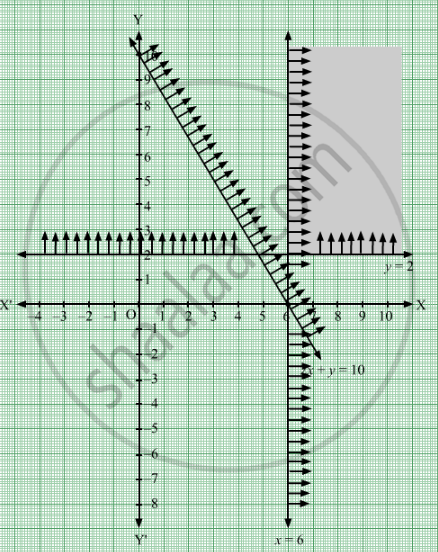Department of Pre-University Education, KarnatakaPUC Karnataka Science Class 12

# Consider a Lpp Given by Minimum Z = 6x + 10y Subjected to X ≥ 6; Y ≥ 2; 2x + Y ≥ 10; X, Y ≥ 0 Redundant Constraints in this Lpp Are (A) X ≥ 0, Y ≥ 0 (B) X ≥ 6, 2x + Y ≥ 10 (C) 2x +Y≥10(D)None of These - Mathematics

MCQ

Consider a LPP given by
Minimum Z = 6x + 10y
Subjected to x ≥ 6; y ≥ 2; 2x + y ≥ 10; xy ≥ 0
Redundant constraints in this LPP are

#### Options

• x ≥ 0, y ≥ 0

• x ≥ 6, 2x + y ≥ 10

•  2x + y ≥ 10

• none of these

#### Solution

$2x + y \geq 10$

We need to minimize the function Z = 6x + 10y
Converting the given inequations into equations, we obtain

$x = 6, y = 2, 2x + y = 10, x = 0, y = 0$

These lines are drawn using a suitable scale
Scale
On X axis
1 Big division = 1 unit
On Y axis
1 Big division = 1 unitThe shaded region represents the feasible region of the given LPP.
We observe that the feasible region is due to the constraint  $x \geq 6, y \geq 2$

So, the redundant constraint is $2x + y \geq 10$

Concept: Introduction of Linear Programming
Is there an error in this question or solution?

#### APPEARS IN

RD Sharma Class 12 Maths
Chapter 30 Linear programming
MCQ | Q 8 | Page 67